# How to Divide in Google Sheets? (Divide Numbers, Columns, Rows, Multiple Cells)

Google Sheets is one the most popular software when it comes to collaborating with multiple users and performing mathematical calculations. The division is one of the most important basic mathematical operations with the help of which we can perform various mathematical operations.

## How to Divide Cells in Google Sheets?

There are two methods with the help of which we can divide cells in Google Sheets and those methods are:

• Using DIVIDE Function
• Using Divide Operator

### How to Divide Columns using the DIVIDE Function?

The steps to divide two cells using the DIVIDE Function are explained below:

• Step 1: Open the Google Spreadsheet where you want to perform the divide operation.
• Step 2: Now in Cell 1, enter the Dividend.
• Step 3: In Cell 2, enter the Divisor.
• Step 4: Now move to cell 3 and simply enter the formula =DIVIDE(A2,B2).
• Step 5: Press the “Enter” button and you will see results as shown below.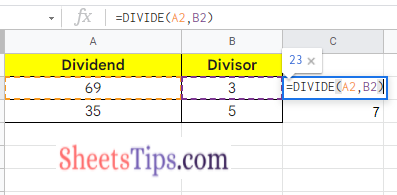Instead of typing the DIVIDE function manually, you can simply click on the Functions icon to enable the DIVIDE function. The steps to get this done are explained below:

1. In the menubar, click on the “Functions” icon.
2. Now from the drop-down window, click on the “All” option. Again a sub-menu will open on the screen.
3. In the submenu, choose “DIVIDE” and that’s it. You can start dividing the dividend by division.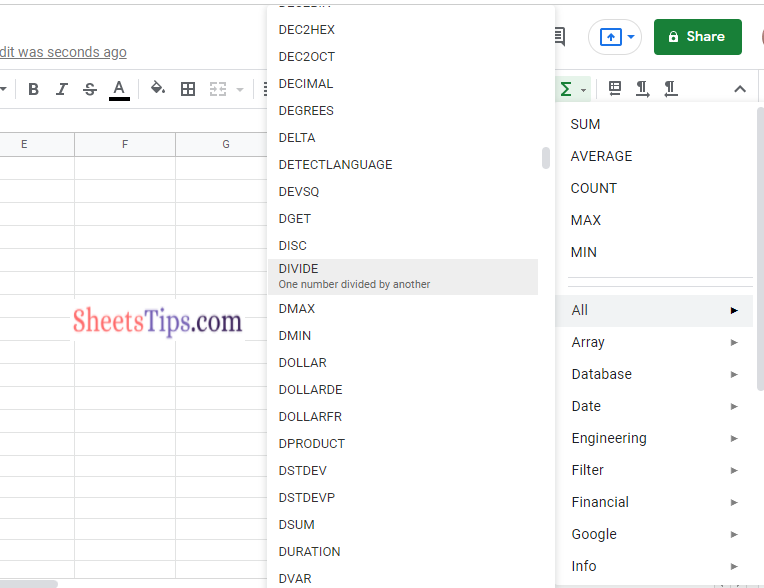### How to Divide Columns using the DIVIDE Operator?

In the previous example, we saw how to divide two columns using the DIVIDE Function. Now in this section let us discuss how to divide two columns using Divide Operator.

• Step 1: Open the Google Sheets where you want to perform Division using the Divide operator
• Step 2: Enter the Dividend in Cell 1.
• Step 3: Move to Cell 2 and enter the Divisor.
• Step 4: Now in Cell 3, enter the formula =(A2/B2).
• Step 5: Press the “Enter” key and you will see the results as shown below.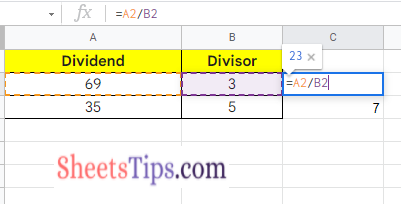Since there is no divide symbol in Google Sheets, we are supposed to use the Slash symbol.

### How to Divide Numbers in Google Sheets?

In the previous section, we discussed how to divide cells in Google Sheets. In this section let us discuss how to divide numbers in Google Spreadsheets:

• Step 1: Open the Google Sheets where you want to divide two numbers.
• Step 2: Now to divide using the DIVIDE function enter the formula =DIVIDE(Dividend, Divisor).
• Step 3: Press the “Enter” button and you will see the results as shown below.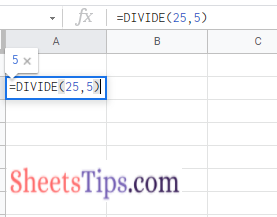Alternatively, we can also use the Divide operator to divide the cells in Google Sheets. The steps divide two numbers using the Divide operator in Google Spreadsheet are briefed below:

• Step 1: Open the Google Sheets to perform Division operations using Divide Operator.
• Step 2: Now move the cell where you want to perform the Division operation.
• Step 3: Enter the formula =Dividend/Divisor.
• Step 4: Press the Return key and you will see the results as shown below.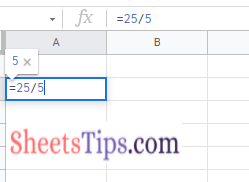### How to Divide Two Rows or Columns in Google Sheets?

With the help of Array Formula, we can divide two or more columns and rows in the Google Spreadsheet. The steps to divide two or more rows and columns using Array Formula are explained below:

• Step 1: Open the Google Spreadsheet where you want to perform Divison.
• Step 2: Now enter the dividend in Cell A.
• Step 3: Enter the Divisors in Cell B.
• Step 4: Go the cell C and enter the formula =ArrayFormula(A2:A5/B2:B5).
• Step 5: Press the “Enter” button and you will see the results as shown below.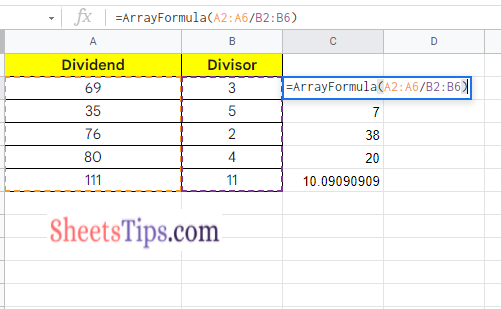Usually, we won’t use Google Sheets to perform simple divisions. We can easily perform simple division using calculators or mobiles. However, if you want to perform a division operation for a huge set of numbers then we can simply adapt the above Array Formula division method.